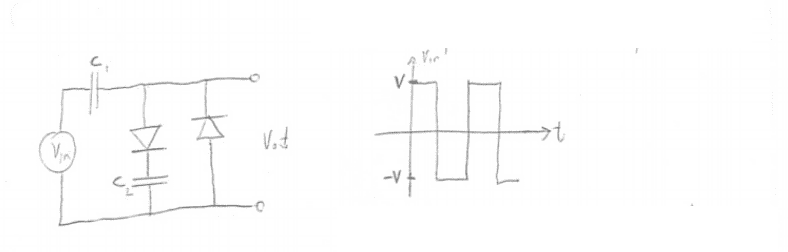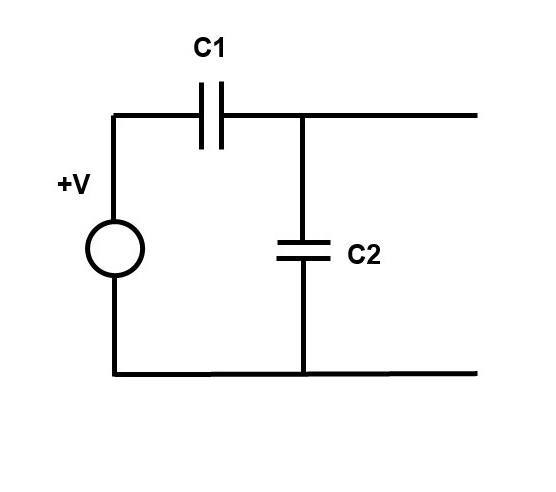# Diode circuit with AC excitation source

• Engineering
Gbox

## Homework Statement

Graph ##V_{out}#### The Attempt at a Solution

When ##V_{in}=V## C1 is positive on the left and negative on the right, and C2 is negative on the the down side and positive on the upper side so there is no Vout?

Homework Helper
Gold Member
When Vin=VVin=VV_{in}=V C1 is positive on the left and negative on the right, and C2 is negative on the the down side and positive on the upper side so there is no Vout?

That's not correct.

For the moment lets assume that V is much greater than the forward voltage of the diode...

When Vin = +V the right hand diode is reverse biased (not conducting) so that can be temporarily removed. The left hand diode is forward biased (conducting) so replace it with a wire. Redraw the circuit to show this.

Then... Are you familiar with the "Potential Divider" circuit?

Gbox
That's not correct.

For the moment lets assume that V is much greater than the forward voltage of the diode...

When Vin = +V the right hand diode is reverse biased (not conducting) so that can be temporarily removed. The left hand diode is forward biased (conducting) so replace it with a wire. Redraw the circuit to show this.

Then... Are you familiar with the "Potential Divider" circuit?
Why is the left hand diode is conducting? the capacitors do not affect the voltage? do not they both charge to the same voltage and the diode is not conducting?